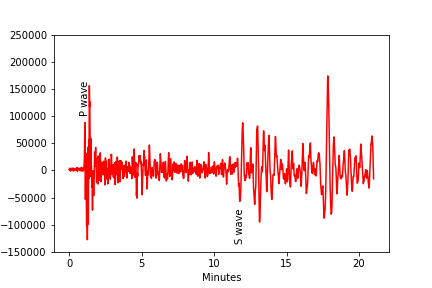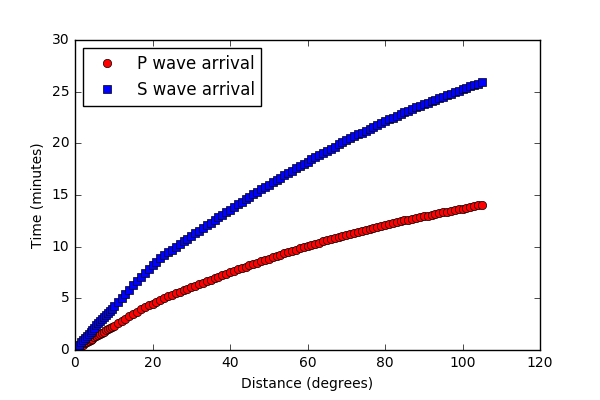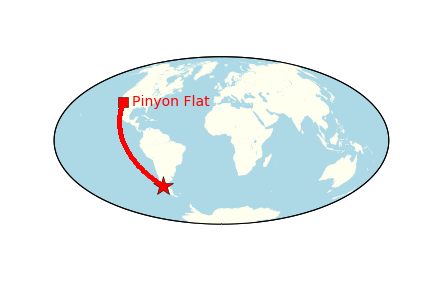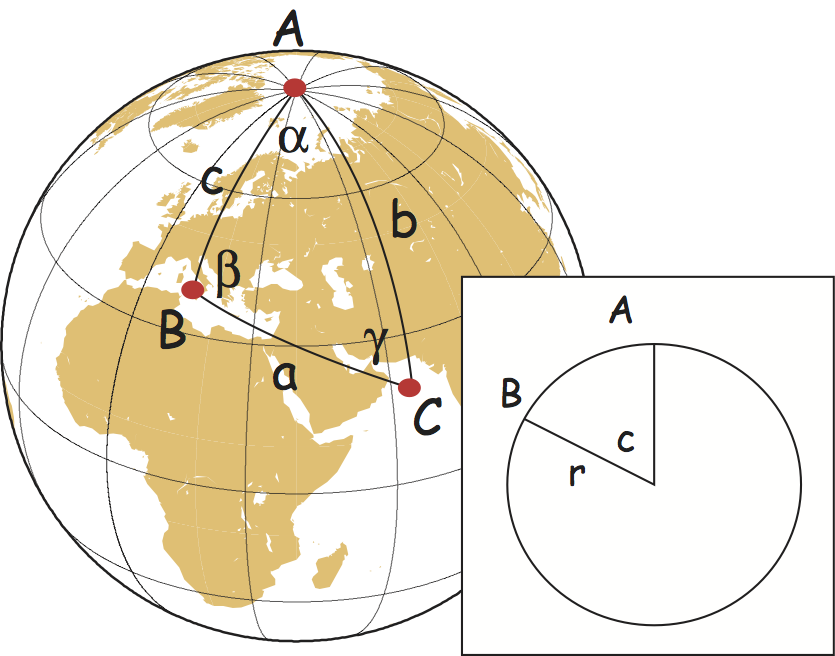In :
import numpy as np
import matplotlib.pyplot as plt
%matplotlib inline
from IPython.display import Image
import pandas as pd
import numpy as np

Lecture 9¶

• Learn how to filter data with Pandas

• Write a program to calculate the great circle distances between two known points.

• Learn how to generate formatted strings for output.

Returning to our original seismogram from Lecture 8 (which we cleverly saved and I moved to the Figures folder):

In :
Image(filename='Figures/seismogram.png') # let's pull this back up.
Out:And we can pick up where we left off by reading in the data which we already converted to minutes and saved in Lecture 8.

In :
Out:
Minutes Velocity
0 0.000000 1807.0
1 0.000833 1749.0
2 0.001667 1694.0
3 0.002500 1618.0
4 0.003333 1516.0

Filtering data with Pandas¶

Considering our seismic record, you know that the P wave arrives in the first few minutes of the record. To find the exact time, we can filter the data to look for the maximum (positive or negative) velocity between two time intervals, say 1 and 1.37 minutes, and take this as the P-wave arrival time.

To look only at the interval between two times, we first need to understand some more about Pandas data structures. Remember that the DataFrames are made up of columns of data (with the indices) and each Column is itself a Series.

We can access a particular Series in two ways. One is to use the Series name as a key (like in Dictionaries).

In :
# I'm using head, because this is a really long series!
0    0.000000
1    0.000833
2    0.001667
3    0.002500
4    0.003333
Name: Minutes, dtype: float64

OR, we can use the Series name as an attribute of the DataFrame:

In :
0    0.000000
1    0.000833
2    0.001667
3    0.002500
4    0.003333
Name: Minutes, dtype: float64

As you can see below, both of these are identical and are instances of a Pandas Series class:

In :
print (type(EQ['Minutes']))
print (type(EQ.Minutes))
<class 'pandas.core.series.Series'>
<class 'pandas.core.series.Series'>

Although I said that these two ways of accessing Series were identical, they are really only identical if there are no spaces in the column name. If you (like most Excel users) like to put spaces in your column names, then you can only use the first form.

Let's take a closer look at one of these Series:

In :
Out:
0    0.000000
1    0.000833
2    0.001667
3    0.002500
4    0.003333
Name: Minutes, dtype: float64

The EQ.Minutes Series are floating point numbers but have indices (starting with 0) so they are like arrays and lists, but are not the same thing. No worries - you can turn a Series into an array with this command (I'm just printing the first 10 elements...):

In :
EQ_array=EQ.Minutes.values
print (EQ_array[0:10])
[0.         0.00083333 0.00166667 0.0025     0.00333333 0.00416667
0.005      0.00583333 0.00666667 0.0075    ]

Or we can make it a list:

In :
EQ_list=EQ.Minutes.tolist()
print (EQ_list[0:10])
[0.0, 0.0008333333333333334, 0.0016666666666666668, 0.0025, 0.0033333333333333327, 0.0041666666666666675, 0.005, 0.0058333333333333345, 0.006666666666666667, 0.0075]

You can filter the EQ DataFrame by placing conditions (which evaluate to True or False) on one of the Series (Minutes or Velocity).

The simplest syntax of a basic Pandas filter is:

DataFrame.Series[condition]

The "P wave arrival" is by definition, the first wave recorded that exceeds "noise". So we need to calculate what the "noise" level is by getting the maximum and minimum values in the first part of the record, say the first minute.

To find all the records where the series EQ.Minutes is less than 1, we need to set the condition to:

EQ.Minutes<1

Let's just see what that does:

In :
EQ.Minutes<1
Out:
0         True
1         True
2         True
3         True
4         True
5         True
6         True
7         True
8         True
9         True
10        True
11        True
12        True
13        True
14        True
15        True
16        True
17        True
18        True
19        True
20        True
21        True
22        True
23        True
24        True
25        True
26        True
27        True
28        True
29        True
...
25170    False
25171    False
25172    False
25173    False
25174    False
25175    False
25176    False
25177    False
25178    False
25179    False
25180    False
25181    False
25182    False
25183    False
25184    False
25185    False
25186    False
25187    False
25188    False
25189    False
25190    False
25191    False
25192    False
25193    False
25194    False
25195    False
25196    False
25197    False
25198    False
25199    False
Name: Minutes, Length: 25200, dtype: bool

So the EQ.Minutes<1 condition returned another Pandas Series with the EQ.Minutes Series being evaluated for each element in the Series.

If we then use the returned set of booleans as the "key" in the DataFrame EQ, Pandas will return all the indices in the DataFrame for which the condition is True:

In :
EQ[EQ.Minutes<1]
Out:
Minutes Velocity
0 0.000000 1807.0
1 0.000833 1749.0
2 0.001667 1694.0
3 0.002500 1618.0
4 0.003333 1516.0
5 0.004167 1394.0
6 0.005000 1282.0
7 0.005833 1198.0
8 0.006667 1077.0
9 0.007500 957.0
10 0.008333 856.0
11 0.009167 745.0
12 0.010000 639.0
13 0.010833 588.0
14 0.011667 533.0
15 0.012500 500.0
16 0.013333 486.0
17 0.014167 494.0
18 0.015000 558.0
19 0.015833 587.0
20 0.016667 699.0
21 0.017500 827.0
22 0.018333 925.0
23 0.019167 1105.0
24 0.020000 1286.0
25 0.020833 1464.0
26 0.021667 1661.0
27 0.022500 1871.0
28 0.023333 2070.0
29 0.024167 2242.0
... ... ...
1170 0.975000 3911.0
1171 0.975833 3916.0
1172 0.976667 3913.0
1173 0.977500 3918.0
1174 0.978333 3923.0
1175 0.979167 3936.0
1176 0.980000 3916.0
1177 0.980833 3921.0
1178 0.981667 3891.0
1179 0.982500 3879.0
1180 0.983333 3839.0
1181 0.984167 3786.0
1182 0.985000 3796.0
1183 0.985833 3739.0
1184 0.986667 3694.0
1185 0.987500 3630.0
1186 0.988333 3574.0
1187 0.989167 3532.0
1188 0.990000 3460.0
1189 0.990833 3384.0
1190 0.991667 3303.0
1191 0.992500 3215.0
1192 0.993333 3109.0
1193 0.994167 3021.0
1194 0.995000 2915.0
1195 0.995833 2857.0
1196 0.996667 2761.0
1197 0.997500 2671.0
1198 0.998333 2627.0
1199 0.999167 2532.0

1200 rows × 2 columns

Notice how this is the same as our masking and filtering efforts with Numpy arrays that you already saw.

Now we assign this new DataFrame to Noise:

In :
Noise=EQ[EQ.Minutes<1]
print (Noise.tail())
Minutes  Velocity
0  0.000000    1807.0
1  0.000833    1749.0
2  0.001667    1694.0
3  0.002500    1618.0
4  0.003333    1516.0
Minutes  Velocity
1195  0.995833    2857.0
1196  0.996667    2761.0
1197  0.997500    2671.0
1198  0.998333    2627.0
1199  0.999167    2532.0

Here we created a NEW DataFrame, Noise, that is a subset of the original EQ and contains only the first minute worth of data.

There are many methods for Panda Series. Two of these are max( ) and min( ). To get the maximum and minimum values of the velocity Series in the first minute, we just apply max( ) and min( ) to the Series:

In :
NoiseMax=Noise.Velocity.max()
print ('maximum value: ',NoiseMax)
NoiseMin=Noise.Velocity.min()
print ('minimum value: ',NoiseMin)
maximum value:  5912.0
minimum value:  -1848.0

Now we can find the first velocity that exceeds (in the absolute sense) NoiseMax in the EQ DataFrame.

To do this, we can find the first peaks or troughs in the Velocity Series that are outside the bounds of NoiseMax and NoiseMin respectively. So, here are all the peaks and troughs in the data series that exceed the noise bounds:

In :
Peaks=EQ[EQ.Velocity>NoiseMax]
Troughs=EQ[EQ.Velocity<NoiseMin]
Minutes  Velocity
1230  1.025000    6344.0
1231  1.025833    6991.0
1232  1.026667    7655.0
1233  1.027500    8309.0
1234  1.028333    8912.0
Minutes  Velocity
1323  1.102500   -4090.0
1324  1.103333   -8919.0
1325  1.104167  -13595.0
1326  1.105000  -18138.0
1327  1.105833  -22528.0

We can get these both in one go by using the Pandas "or" trick, which has the general syntax of:

DataFrame.Series[(condition_1) | (condition_2) ]

where the '|' stands for 'or'.

While we are on the topic, other types of conditions, including 'and' and 'not'. 'and' is represented by a '&':

DataFrame.Series[(condition_1) & (condition_2) ]

which requires both conditions to be True, and "and not" is a '& ~':

DataFrame.Series[(condition_1) & ~(condition_2) ]

which requires condition_1 to be True and condition_2 to be False.

Anyway, we can get a single data frame with all the values exceeding bounds (both negative and positive) this way:

In :
PeaksandTroughs=EQ[(EQ.Velocity>NoiseMax)|(EQ.Velocity<NoiseMin)]
Minutes  Velocity
1230  1.025000    6344.0
1231  1.025833    6991.0
1232  1.026667    7655.0
1233  1.027500    8309.0
1234  1.028333    8912.0

So the first Velocity that exceeds the noise is a peak that arrives at 1.025 minutes.

We can make a variable named PwaveArrival by converting the Series PeaksandTroughs.Minutes to an array (by using the .values method on the Series) and then selecting the first value in this array.

In :
PwaveArrival=PeaksandTroughs.Minutes.values
PwaveArrival
Out:
1.025
In :
Image(filename='Figures/seismogram.png') # let's pull this back up.
Out:After the P wave arrival, the earthquake rumbles along for a few minutes, so we need to re-characterize the noise floor, say between 5 and 10 minutes. Let's we repeat the noise exercise for the period between 5 and 10 minutes. In this case, we have TWO conditions: the first being Minutes$>$5 and the second being Minutes$<$10.

To impose TWO conditions, we can combine each (enclosed in parentheses) with '&':

In :
Noise2=EQ[(EQ.Minutes >5) & (EQ.Minutes<10)]
print (Noise2.head()) # see, we only have data after 5 minutes
print (Noise2.tail()) # and before 10 minutes.
Minutes  Velocity
6001  5.000833  -15898.0
6002  5.001667  -17112.0
6003  5.002500  -18312.0
6004  5.003333  -19544.0
6005  5.004167  -20811.0
Minutes  Velocity
11995  9.995833    9719.0
11996  9.996667   10343.0
11997  9.997500   10992.0
11998  9.998333   11599.0
11999  9.999167   12151.0

Following the same logic as before, we can find the bounds for the noise:

In :
Noise2Max=Noise2.Velocity.max()
Noise2Min=Noise2.Velocity.min()
print (Noise2Max,Noise2Min)
46816.0 -44753.0

To find the new S wave arrival, we find the peaks and troughs that exceed the maximum and minimum values in the time between 5 and 10 minutes. First we need to filter for data after the first 10 minutes.

In :
PostP=EQ[EQ.Minutes>10]

Now we can use our handy 'or' operator '|' like this:

In :
PeaksandTroughs2=PostP[(PostP.Velocity>Noise2Max)|(PostP.Velocity<Noise2Min)]
Minutes  Velocity
14089  11.740833  -44843.0
14090  11.741667  -45729.0
14091  11.742500  -46570.0
14092  11.743333  -47369.0
14093  11.744167  -48142.0

The S wave arrives as a trough at about 11.7 minutes into the earthquake.

Let's retrieve that value like we did before.

In :
SwaveArrival=PeaksandTroughs2.Minutes.values
SwaveArrival
Out:
11.740833333333333

So the time delay between P and S wave arrivals can be calculated by the difference in their arrival times:

In :
delayTime= SwaveArrival-PwaveArrival
delayTime
Out:
10.715833333333332

From this we see that there was a 10.7 minute delay.

If you have already taken geophysics, you probably know that seismologists have figured out the velocity of various seismic waves as a function of depth in the Earth. From this model, there is a basic look up table that specifies the delay times as a function of distance (expressed as an angle) between the source and the receiver. This model is in the file DeltaTimeData.csv that we already looked at, munched on and saved in Lecture 8.

Let's take another look at this file:

In :
Out:
Degrees P_wave_minutes P_wave_seconds S-P_minutes S-P_seconds P_decimal_minutes SP_decimal_minutes S_decimal_minutes
0 0.0 0 5.4 0 4.0 0.090000 0.066667 0.156667
1 0.5 0 10.6 0 7.8 0.176667 0.130000 0.306667
2 1.0 0 17.7 0 13.5 0.295000 0.225000 0.520000
3 1.5 0 24.6 0 19.0 0.410000 0.316667 0.726667
4 2.0 0 31.4 0 24.4 0.523333 0.406667 0.930000

So, how many degrees away from the source is the reciever based on the DeltaTimeData data from the Reference Earth Model?

Here is the plot from our reference model (again).

In :
Image(filename='Figures/TravelTime.png')
Out:We can bracket the angular distance this represents by filtering the DeltaTimeData DataFrame for those records within, say, .2 minutes of our delay time:

In :
find_delta=DeltaTimeData[
(DeltaTimeData.SP_decimal_minutes>delayTime-.2) &
(DeltaTimeData.SP_decimal_minutes<delayTime+.2)]
find_delta  # find_delta has all the records with SP_min less than 10
Out:
Degrees P_wave_minutes P_wave_seconds S-P_minutes S-P_seconds P_decimal_minutes SP_decimal_minutes S_decimal_minutes
96 86.0 12 37.0 10 31.9 12.616667 10.531667 23.148333
97 87.0 12 41.9 10 36.7 12.698333 10.611667 23.310000
98 88.0 12 46.7 10 41.4 12.778333 10.690000 23.468333
99 89.0 12 51.4 10 46.1 12.856667 10.768333 23.625000
100 90.0 12 56.1 10 50.7 12.935000 10.845000 23.780000

So according the model, the earthquake occurred around 89 degrees away from the reciever.

We know the location of both the earthquake and the reciever, so we could calculate the actual separation. Let's do that now.

Great Circle Distances¶

The earthquake occured in Chile and was recorded at Pinyon Flats (near us).

Aside: we will learn how to generate this map in the coming lectures!

In :
Image(filename='Figures/greatCirc.png')
Out:First, we must calculate the angular distance (great circle distance in arc length - not kilometers) for the two points, B and C.

We'll need to use spherical trigonometry which is very useful in Earth Science because we live on a sphere.

In :
Image(filename='Figures/strig.png',width=500,height=500)
Out:[Figure from Tauxe et al., 2010, Essentials of Paleomagnetism; https://earthref.org/MagIC/books/Tauxe/Essentials/WebBook3ap1.html#x20-211000A.3 ]

One of the laws of spherical trigonometry is the Law of Cosines:

$\cos a = \cos b \cos c + \sin b \sin c \cos \alpha$.

In our case, $a$ is the great circle distance between points on the globe, $B$ and $C$. Well, well, well, that could come in handy here. So to get $a$ we need to know $b, c$ and $\alpha$. $b$ and $c$ are the co-latitudes (90-latitude) of points B and C, respectively, and $\alpha$ is the difference in the two longitudes.

Now we have a formula, we can write a function to calculate the great circle distance from the latitudes and longitudes of the two points (lat_1,lon_1,lat_2,lon_2).

In :
def great_circle(lat_1,lon_1,lat_2,lon_2):
"""
calculates great circle distance between two points on a globe
Parameters:
lat_1 : float
latitude of first point
lon_1 : float
longitude of first point
lat_2 : float
latitude of second point
lon_2 : float
longitude of second point
Returns:
a : float
great circle distance in degrees
"""
# first we have to convert the latitudes to colatitudes:
colat_1,colat_2=90.-lat_1,90.-lat_2
# and alpha is the difference betwee the two longitudes
alpha=lon_2-lon_1
# Then lets make life easy on us and convert degrees to radians
# from spherical trig we know that:
cosa=np.cos(colat_1)*np.cos(colat_2)+np.sin(colat_1)*np.sin(colat_2)*np.cos(alpha)
# solve for a
a=np.arccos(cosa)# take the arc cosine of cosa
# remember to convert back to degrees!
return np.degrees(a) # return the great circle distance in degrees.
In :
PF_lat,PF_lon=33.3,-115.7
EQ_lat,EQ_lon=-43.42,-73.95
Delta=great_circle(PF_lat,PF_lon,EQ_lat,EQ_lon)
print ('Degrees separation between source and receiver: ',Delta)
Degrees separation between source and receiver:  85.66731577526346

Earlier, we guessed that it would be about 89 degrees. So this is pretty close given our quick and dirty guesstimate.

Formatting strings in Python¶

Now for a word about formatting strings. Notice how the output of the above print statement printed out all the decimal places. We can do better! To show only the first decimal place we can use string formatting.

The structure of a formatting statement is:

'%FMT'%(DATA),

where FMT is a 'format string' and DATA is the variable name whose value we want to format. Here is an example in which FMT is:

3.1f.

The first number (3) is the number of characters in the output. The second number (1) is the number of characters AFTER the decimal place. The 'f' means that DATA is a floating point variable.

Other format strings include: %s for a string, %i for an integer, %e for 'scientific notation'.

In :
print ('no formatting: ',Delta) # no formatting
print ('formatted: ','%3.1f'%(Delta)) # with formatting

# or can use round(Delta,1)
print ('rounded: ',round(Delta,1))
no formatting:  85.66731577526346
formatted:  85.7
rounded:  85.7

Recall from Lecture 2:

In :
number=1 # an integer
Number=1.0 # a floating point
NUMBER='1' # a string

we can use the formatted string trick on them like this:

In :
print ('%i'%(number))
print ('%f'%(Number))
print ('%3.1f'%(Number))
print ('%s'%(NUMBER))
1
1.000000
1.0
1

Or for a bigger number:

In :
bignum=np.pi*1000
print (bignum)
print ('%6.5e'%(bignum))
print ('%3.2e'%(bignum))
3141.592653589793
3.14159e+03
3.14e+03

Assignment #3¶

Your code must be fully commented, the notebook must have the correct name and it must be turned in to Canvas before the deadline.

To turn it in, you must include the datafile you downloaded in a zip file with your solution notebook.

In [ ]: